Jul 24

## World Changing Mathematical Discoveries

Although mathematics is not the most attractive field of study nowadays, there were some days when it was quite appealing. Millions of students and great illuminated minds dedicated their life to make discoveries that eventually improved quantity, structure, space and change – the main concepts studied by mathematics. It started as a philosophy, developed as a science and finally influenced everything, from technology and architecture to art. While most of us don’t realize it, the founding and evolution of mathematics is the main reason for the modern life we take for granted.

The Egyptian decimal system and the Mesopotamian weights and measures
While the ancient Greek civilization is considered the founder of the main principle of mathematics, archaeologists found proofs that Egyptians also developed quite an advanced decimal system. This is the earliest system that allowed indefinite counting through adding new symbols. The Egyptian hieroglyphs reveal that the system is in evidence since around 3000 BC. This innovative model influenced the Minoans’ own decimal system, a Bronze Age civilization that lived on island of Crete.
While de Egyptians early mathematicians were focused on the decimal system, the Mesopotamian scientists developed a functional weighting and measuring system sometime around 4000 BC. Sexagesimal schemes (a numeral system that has 60 as its base) were used to count slaves, animals, fish, dead animals, certain types of beer and milk products. Other innovative patterns were created to count field measurement, wheat, malt, milk and beer measurement.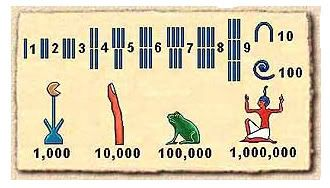Source: https://content.ncetm.org.uk/images/microsites/primary_magazine/issue_4/egyptian_2.jpg

Pythagoras’ findings in geometry, irrationality and the square root of two
Pythagoras of Samos was an Ionian Greek philosopher and mathematician that among other, founded a religious movement called Pythagoreanism. He lived between 570 – 495 BC, a period when he founded the most famous ancient school of mathematics. The Pythagoreans thought that mathematics is not just an advanced subject, but the base on which relies the principles of all the surrounding things. Pythagoras has commonly been given credit for discovering a great geometrical theorem that states that in a right-angled triangle area, the area of the square on the hypotenuse is equal to the areas of the squares of the other sides. Due to the secrecy that surrounded the Pythagorean School, there is no evidence that Pythagoras itself has worked on this theorem.
This theorem however, pushed Hippasus, one of the Pythagorean students, to discover the existence of irrational numbers. When trying to represent the square root of 2 as a fraction, using geometry, he proved that one cannot write the square root of 2 as a fraction, therefore this was irrational. His finding could not be accepted by his fellow Pythagoreans, therefore he was ultimately thrown overboard and drowned.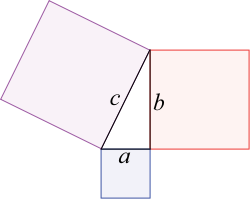The findings on the negative numbers
Although the recordings claim that Heron of Alexandria (an ancient Greek mathematician and engineer that lived in the famous Egyptian city) was the first to mention the existence of negative numbers, there are no actual findings that his work was however related to this concept. Brahmagupta was the first scientist ever to have developed advanced rules of dealing with the negative numbers. He was an Indian astronomer and mathematician that lived between 598 – 668 CE. His main work is Brāhmasphuṭasiddhānta (The Correctly Established Doctrine of Brahma), in which he cultivates some extraordinarily advanced ideas such as the mathematic role of zero, rules for manipulating both the negative and positive numbers, computing square roots, solving linear and quadratic equations and many more.
And the most interesting thing of all is that the book was completely written in verse, making it not only a milestone writing in mathematics, but also a wonderful piece of literature.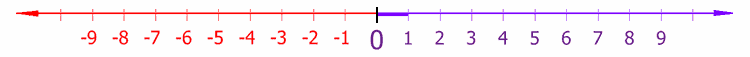The Arabic numerals
These are the ten digits every child is learning today and everyone is using day by day, in the vast majority of the world. The Arabic numerals, or the Hindu-Arabic numerals were developed by Indian mathematicians and their main feature is that a sequence of numbers such as “123” is read as a whole number. Adopted by the Islamic mathematicians, they passed to the Arabs in west, which transmitted them to Europe, in the Middle Ages. The Europeans spread them in the world, throughout trading, books and colonialism.
The digits 1 to 9 evolved from the Brahmi numerals, and Indian numeral system developed in the 3rd century BCE. The first universally accepted inscription that contains the use of the 0 glyph dates back from the 9th century and it was discovered at Gwalior, in Central India. Around the year 1000, Gerbert of Aurillac (later known as Pope Sylvester II) used his position to spread the knowledge of numerals in Europe. Still, the most active early promoter of the Hindu-Arabic numerals was Fibonacci, a mathematician born in the Republic of Pisa that eventually became famous for the Fibonacci sequence.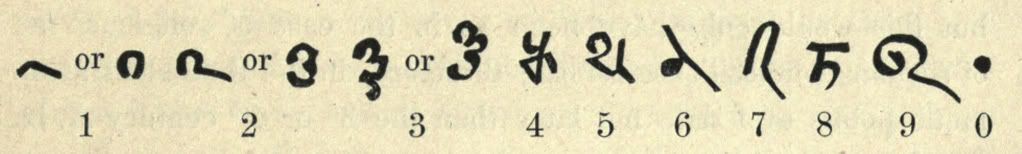Symbols for basic mathematical operations
Nicole Oresme was one of the greatest philosophers in the late Middle Ages. His published writings included influential works on economics, mathematics, physics, astronomy, philosophy and theology. Although his profile was not mathematic by default, he is considered the father of the modern symbols for subtraction (“-“) and addition (“+”), used first sometime around 1360. These, along with other significant discoveries in mathematics are contained in Tractatus de configurationibus qualitatum et motuum.
Almost two centuries later, the modern equals sign (“=”) is mentioned for the first time in The Whetstone of Witte, published in 1557. It was written by Robert Recorde, a Welsh physician and mathematician. The Whetstone of Witte was also the first book in English to contain the plus and minus signs.
More than half of century will have to pass until the first use of the multiplication sign (“x”). This happened in 1618, and the main “responsible” for this innovation was William Oughtred, an English mathematician. Moreover, this early genius in mathematics introduced for the first time the abbreviations “sin” and “cos” for the sine and cosine functions.
Finally, the modern division symbol (“÷”) was introduced by Johann Rahn, a Swiss mathematician. The sign firstly appeared in Teutsche Algebra, a work published in 1659.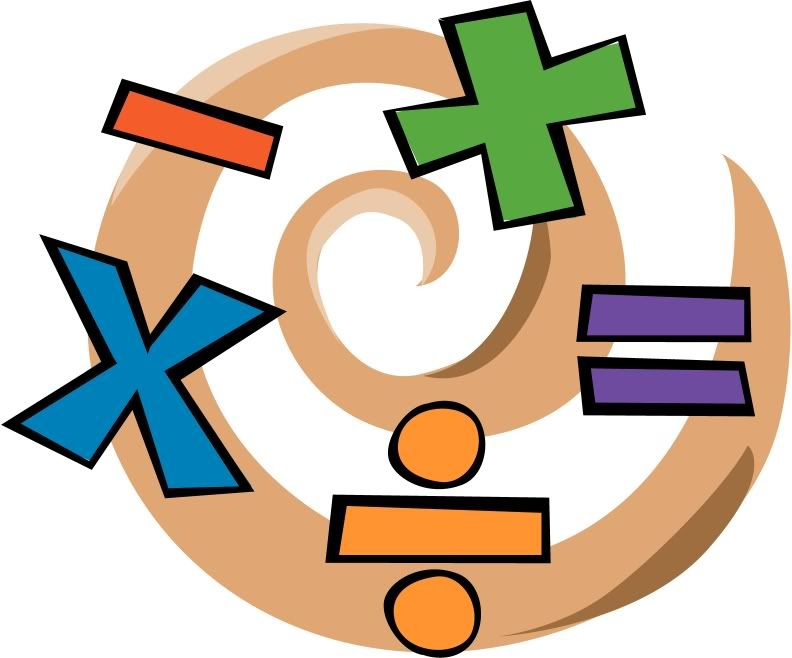Source: http://www.mrs-brunell.org/images/Math%20icons/math_symbol_clipart.jpg

Other 16th-17th century mathematical discoveries that marked the evolution of mathematics
In the 16th-17th century, England had numerous illuminated minds in the field of mathematics, their work being acknowledged as a great contribution to the progress in mathematics. John Wallis was one of the great mathematicians that lived in that period and who was given a partial credit for the infinitesimal calculus. On the other hand, he is fully credited with introducing the symbol for infinity, in 1655: “”.
Although the letter “π” (pi) exists since the ancient Greek alphabet was created, its use in mathematics was proposed by William Jones in 1706, to represent a constant that equals the ratio of the circumference of a circle to its diameter.
Leonhard Euler was a pioneering Swiss mathematician and physicist renowned for its exquisite work in infinite calculus, graph theory and mathematical analysis. He lived most of his life in St. Petersburg (Russia) and Berlin (Prussia), where he managed to print all his contributions that would eventually occupy somewhere between 60 and 80 quarto volumes. Two mathematical notations were introduced and popularized through his work – the letter e, for the base of the natural logarithm and the letter i to denote the imaginary unit. Although Jones introduced the usage of the letter “π”, Leonhard Euler was the one that made it popular amongst the mathematicians at that time.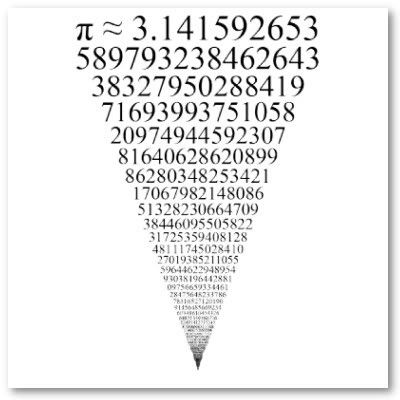Source: http://rlv.zcache.com/the_first_thousand_digits_of_pi_looks_infinite_poster-p228230223011912582t5ta_400.jpg

The hyperbolic geometry
This type of geometry is a non-Euclidean geometry, which infirm the parallel postulate of the Euclidean geometry that states the fact that two parallel lines never intersect. In the hyperbolic geometry, there are an infinite number of lines parallel to a given line.
Developed by Nikolai Lobachevsky (a Russian mathematician) and Janos Bolyai (a Hungarian mathematician that lived in Transylvania), the hyperbolic geometry has no precise analogue of the Euclidean parallel lines and its main characteristic is the fact that the angles of a triangle add to less than a straight angle. The development of this kind of geometry led to a series of discoveries in physics and many other mathematical related domains.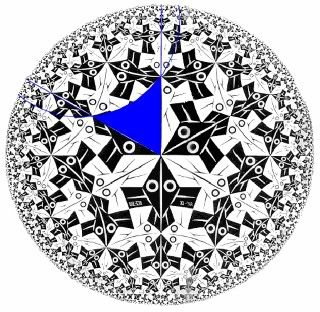Source: http://www.btinternet.com/~connectionsinspace/ESCHERT1.jpg

Einstein’s general relativity
The general theory of relativity is the geometric theory of gravitation published by Albert Einstein in 1916. It currently serves as a description of gravitation in the modern field of physics. Unlike the hyperbolic geometry (which refutes some of the basic principles of “classical” geometry)  , the general relativity generalizes the special relativity findings before and Newton’s universal law of gravitation and provides gravity as a geometric property of space, time or space-time.
General relativity changes the way classical physics are perceived – especially the passing of time, the geometry of space, the motion of bodies in free fall and the propagation of light. It is also the simplest theory that is consistent with experimental data.
The general theory of relativity therefore implies the existence of black holes – regions in space where light and time are distorted in a way that nothing can escape. In addition, general relativity is a basis for the cosmological models that are responsible for the continuous expanding universe.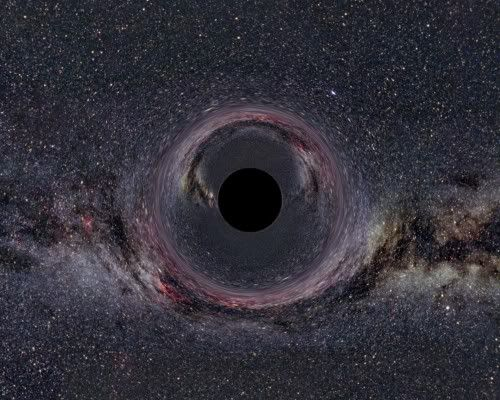Add a comment Emots Enable HTML Enable UBB Enable Emots Hidden Remember Nickname   Password   Optional Site URI   Email   [Register]